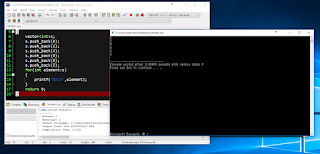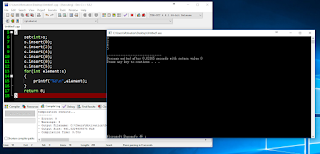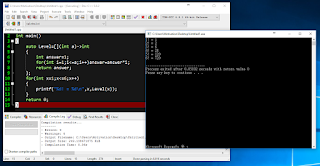## 2016年10月26日 星期三

### 給新手的C++教學 (上冊) - 13 - 25. 「新版C++」－－「C++11」的好用功能

C++看起來已經是一個很完整、功能強大的程式語言了

「auto」的用法:

```#include<cstdio>
int main()
{
double a=8.3;
//double b=a;
auto b=a;//這一行和上面那一行被註解掉的程式碼是等價的
printf("a=%f, b=%f\n",a,b);
return 0;
}```執行結果

```#include<cstdio>
struct DateOfKSHSFairs
{
int month,day;
};
int main()
{
DateOfKSHSFairs day1;
day1.month=10;
day1.day=28;
//DateOfKSHSFairs day2=day1;
auto day2=day1;//這一行和上面那一行被註解掉的程式碼是等價的
day2.day=29;
printf("高雄市立高雄高級中學園遊會\nday1=%d月%d日, day2=%d月%d日\n",day1.month,day1.day,day2.month,day2.day);
return 0;
}```執行結果

「for迴圈」的另類用法：

```#include<cstdio>
int main()
{
int s={0,2,4,0,0,5};
for(int element:s)
{
printf("%d\n",element);
}
return 0;
}```執行結果

vector版本：

```#include<cstdio>
#include<vector>
using namespace std;
int main()
{
vector<int>s;
s.push_back(0);
s.push_back(2);
s.push_back(4);
s.push_back(0);
s.push_back(0);
s.push_back(5);
for(int element:s)
{
printf("%d\n",element);
}
return 0;
}```執行結果

set版本：

```#include<cstdio>
#include<set>
using namespace std;
int main()
{
set<int>s;
s.insert(0);
s.insert(2);
s.insert(4);
s.insert(0);
s.insert(0);
s.insert(5);
for(int element:s)
{
printf("%d\n",element);
}
return 0;
}```執行結果

map版本：

```#include<cstdio>
#include<map>
using namespace std;
int main()
{
map<char,int>s;
s['B']=1;
s['0']=9;
s['5']=9;
s['9']=8;
s['0']=0;
s['2']=4;
s['0']=0;
s['8']=1;
s['3']=1;
//for(pair<char,int> element:s)
for(auto element:s)//這一行和上面那一行被註解掉的程式碼是等價的
/*看到auto了嗎?
電腦將會自動把auto替換為pair<char,int>
當你不知道element要用甚麼型別時特別好用*/
{
printf("key=%c, value=%d\n",element.first,element.second);
}
return 0;
}```執行結果

「匿名函式 (又稱Lambda、匿名運算式)」的用法：

```#include<cstdio>
int main()
{
auto Level=[](int a)->int
{
};
for(int x=1;x<=6;x++)
{
printf("%d! = %d\n",x,Level(x));
}
return 0;
}```執行結果

```#include<cstdio>
int main()
{
for(int x=1;x<=6;x++)
{
printf("%d! = %d\n",x,[](int a)->int
{
}(x));
}
return 0;
}```執行結果

[](int a)->int
{
}

「中括號[]」寫出來放著就好
「小括號()」裡面放入引數 (甚麼是引數?)
「箭頭->」後面接回傳值 (甚麼是回傳值?) 的型別
「大括號{}」裡面放入函式的程式碼

-----The End-----

(版權所有 All copyright reserved)